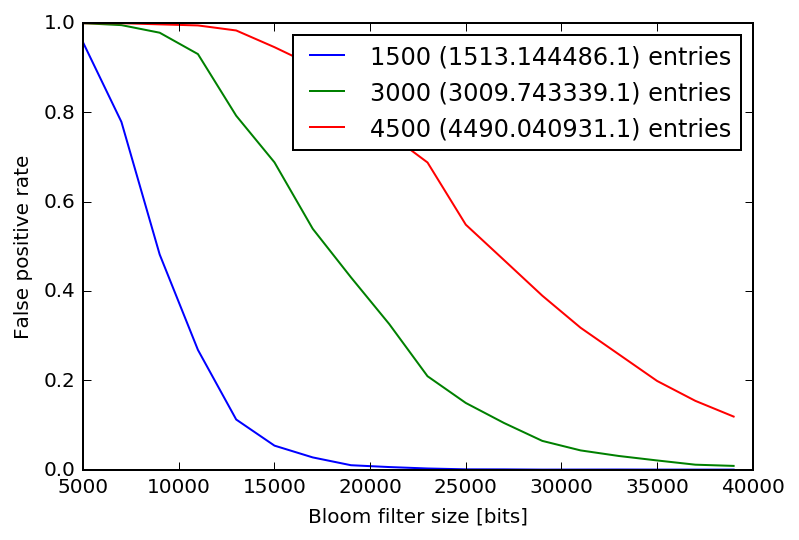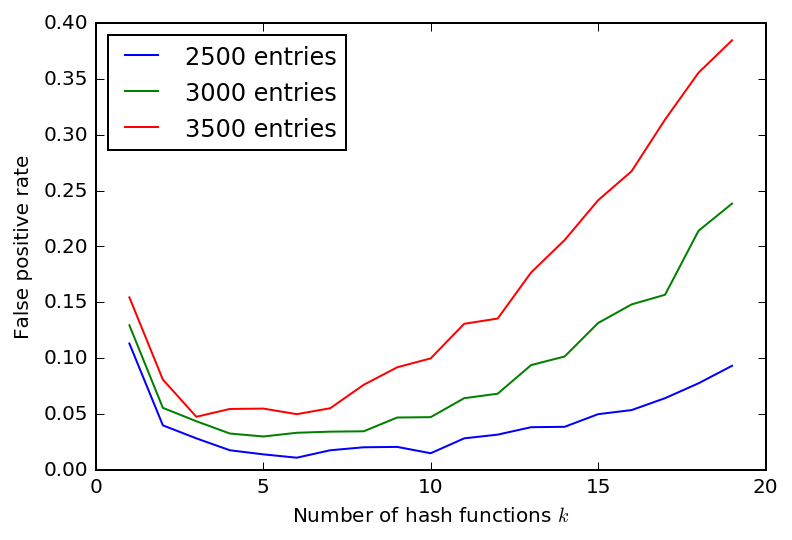# An Improbable Afternoon

Spend an afternoon understanding these seemingly magical algorithms.

This post covers a few probabilistic data structures in python. Each of them deals with counting things in one form or another (how many unique items, is an item present, how many of each have we seen, etc).

The idea behind these probabilistic data structures is that you can use a lot less memory to perform each task if you are happy to get an estimate instead of a precise answer. At first this sounds like it would make your life more difficult, but often it turns out to allow you to do things that were impossible before.

## Call me, ... maybe¶

The theme tune you should be listening to for this post (because the Tour starts in a few days):

In :
from IPython.display import VimeoVideo
VimeoVideo(48756378)

Out:

## Old School Spellchecking¶

A famous example of using probabilistic data structures is the following: How would you implement a (fast) spellchecker if there are more words in your dictionary than you have RAM? In the early days of computers this was the case. The slow solution is to search your on disk dictionary for each word in the document. A faster solution is to be able to quickly rule out words that are definitely not in the dictionary, and only going to disk for the words you think might be in the dictionary. This is what the Bloom filter was invented for.

## Contents¶

These four data structures are definitely covered in this post:

• Bloom filters ✔︎
• Count-min sketch ✔︎
• MinHash (not done yet)
• HyperLogLog ✔︎

Word of warning: I tried to keep the implementations as simple as possible. The goal was to make it as easy to understand what was going on as possible. There are several optimisations you can apply if you want to use these at scale. Please find yourself a good, well tested implementation somewhere else if you want to use these in production.

In :
%config InlineBackend.figure_format='retina'
%matplotlib inline

In :
import random
random.seed(12345)
from collections import Counter
import string

import numpy as np
import matplotlib.pyplot as plt

from sklearn.utils import murmurhash3_32
from sklearn.utils import check_random_state

# python's builtin hash function isn't even approximately random
def hash_(n, seed=1):
return murmurhash3_32(n, seed=seed, positive=True)


## Do I know you?¶

The simplest form of keeping track of items you have seen is to create a list to which you add each new item. The good news is that this is easy to implement, you can check if you have seen X and answer the question "What are all the items you have seen?". The bad news is that you need to have enough memory to store each new, unique item.

Answering the question "Have I seen X before?" does not require you to store every item you have seen though! Instead you can use a list of m bits and some method to convert an item into an index into that list. So when you see X you calculate the position i in the list corresponding to the item and turn on the bit there.

To find out if you have seen X before you calculate the index i and check if the bit there is turned on or off. Simplez.

### Hash functions¶

How to turn an item into an index? A hash function. There are many different hash functions for many different tasks. One thing you can do with them is to "summarise" an item in a single number. For example hash('hello world') -> 6355 and hash('hello world!') -> 2359. We can now use this number as our index.

The eagle-eyed will have spotted a caveat with this approach: What if two different items X and Y end up producing the same index i? This could happen either because their hash is the same or because we only have m entries in our list.

This is why it is called a probabilistic data structure. We summarise items and keep track of counts with a small number of bits. This means we might make a mistake. In thise particular case you can see that increasing m (for a given number of unique items) controls how likely you are to make a mistake. If you make m very small you need hardly any memory and make mistakes all the time. If you make m very large you will use as much as if you stored every item and make nearly no mistakes.

## Multiple hashes¶

It turns out you can do better than using m bits and one hash function without increasing the amount of memory you use. The trick is to use k different hash functions which turn on bits in the same list of length m. To check if an item has been seen you check if all k positions are turned on. If one of them is off you know you did not see this item.

A simple way of understanding why this approach does better than using one hash function is to think of each hash function as a member of a crowd. The reason for the crowd of hash functions doing better than a single one of them is the same why a crowd does better at estimating the weight of a cow. You are averaging several independent estimates.

## The Bloom filter¶

This then is the BloomFilter: an array of m bits and k hash functions. Membership testing without knowing who is a member! Sounds crazy, but this does actually work.

In :
class BloomFilter:
def __init__(self, m, k, random_state=None):
# k hash functions and m bits
self.m = m
self.rng = check_random_state(random_state)
self.bits = np.zeros(m, dtype=bool)
r = self.rng.randint(1e9)
self.k = [r + k for k in range(1, k+1)]

for i in self.k:
idx = hash_(val, i) % self.m
self.bits[idx] = True

def __contains__(self, val):
for i in self.k:
if not self.bits[hash_(val, i) % self.m]:
return False

return True

def entries(self):
"""Approximate number of entries."""
X = np.sum(self.bits)
return -(self.m / float(len(self.k))) * np.log(1 - X/self.m)


Let's take it for a ride. We create a BloomFilter with a memory of 15000 bits and 20 hash functions. Then fill it with 1000 random numbers. Afterwards we check which fraction of the numbers we have seen previously is recognised by the BloomFilter. We also check what fraction of previously unseen numbers are incorrectly assumed to be a member.

In :
b = BloomFilter(15000, 20)

def fill(B, n_test=1000, upper=1e9, exact=False):
if exact:
exact_ = set()

for n in range(n_test):
r = np.random.randint(upper)
if exact:

if exact:
return exact_

exact = fill(b, exact=True)

n_true = 0
for e in exact:
if e in b:
n_true += 1

def fakes(B, n_test=1000, upper=1e9):
n_fake = 0
for e in np.random.randint(upper, size=n_test):
if int(e) in B:
n_fake += 1

return n_fake / n_test

print('True positive rate: %.3f False positive rate: %.3f.' % (n_true / len(exact),
fakes(b)))

True positive rate: 1.000 False positive rate: 0.001.


Below a small illustration how the false positive rate changes with the size of the bloom filter. As expected, the more memory you allocate the smaller the false positive rate. For a given size of bloom filter, the false positive rate gets worse as the number of values stored increases. The legend shows the true number of entries as well as the estimated number of entries in brackets.

The second figure investigates the behaviour as a function of the number of hash functions at fixed bloom filter size.

In :
sizes = np.arange(5000, 40000, step=2000)

for n_test in (1500, 3000, 4500):
fake_rate = []
for size in sizes:
b = BloomFilter(size, 20)
fill(b, n_test=n_test)
fake_rate.append(fakes(b, n_test=10000))

plt.plot(sizes, fake_rate, label='%i (%f.1) entries' % (n_test, b.entries()))

plt.legend(loc='best')
plt.xlabel('Bloom filter size [bits]')
plt.ylabel('False positive rate')

Out:
<matplotlib.text.Text at 0x108ec9940>In :
for n_test in (2500, 3000, 3500):
hash_funs = np.arange(1, 20)
fake_rate = []
for k in hash_funs:
b = BloomFilter(22000, k)
fill(b, n_test=n_test)
fake_rate.append(fakes(b, n_test=3000))

plt.plot(hash_funs, fake_rate, label='%i entries' % n_test)

plt.legend(loc='best')
plt.xlabel('Number of hash functions $k$')
plt.ylabel('False positive rate')

Out:
<matplotlib.text.Text at 0x1090a83c8>## Count-Min Sketch¶

After this deep dive into bloom filters, let's move on to data structures which use bloom filters as building blocks.

One thing you can not do with a standard bloom filter is count how often you have seen an item. A count-min sketch is similar to a bloom filter, except it can estimate how often it has seen each item, not just answer yes or no.

Instead of having just one array which you index with $k$ hash functions you create $d$ arrays of size $w$, and index each with its own hash function. To find the number of times you have seen an item you take the minimum count over all arrays.

In :
class CountMinSketch:
def __init__(self, w, d, random_state=None):
self.rng = check_random_state(random_state)
self.w = w # cols
self.d = d # rows

self.k = self.rng.randint(1e9, size=d)
self.t = np.zeros((d, w), dtype=np.int)
self.N = 0
self.hash_ = np.vectorize(hash_)

def update(self, val):
idx = self.hash_(val, self.k) % self.w
self.t[np.arange(len(self.t)), idx] += 1
self.N += 1

def query(self, val):
idx = self.hash_(val, self.k) % self.w
return np.min(self.t[np.arange(len(self.t)), idx])


The code below stores 1000 unique words in a count min sketch with counts for each word varying between zero and 50. Afterwards we print out the true and the estimated counts.

In :
cms = CountMinSketch(100, 300)

words = {}
for n in range(1000):
word = ''.join([random.choice(string.ascii_lowercase) for _ in range(10)])
count = np.random.randint(50)
words[word] = count
for _ in range(count):
cms.update(word)

for n,word in enumerate(words):
print(words[word], cms.query(word))
if n > 20:
break

5 64
49 113
31 92
46 92
1 65
35 61
38 57
15 38
17 70
1 49
14 19
8 12
45 70
15 16
44 96
17 83
14 62
10 48
44 71
10 40
12 64
38 110


## HyperLogLog¶

Next up: How many distinct items are there in a stream of data? The HyperLogLog (what a cool name!) can help you with that.

The implementation is a bit more involved. Mostly because you need to make a few corrections for edge cases (few and very many items).

The intuitive explanation of how and why the HyperLogLog works goes like this: By keeping track of the longest run of heads someone has flipped you can estimate how often they must have flipped the coin. The more often someone flips a coin in a row, the higher the probability for them to observe a long run of heads.

In :
def binary(w):
"""Binary representation of w"""
return bin(w)[2:]

def rho(bit_string):
"""Index of first 1 in bit_string"""
for n,x in enumerate(reversed(bit_string)):
if x == '1':
return n+1

return 32

def alpha(b):
if not (4 <= b <= 16):
raise ValueError("b=%d should be in range [4 : 16]" % b)

if b == 4:
return 0.673

if b == 5:
return 0.697

if b == 6:
return 0.709

return 0.7213 / (1.0 + 1.079 / (1 << b))

def hyperloglog(items, b=4):
"""Approximate count of distinct elements in items"""
m = 2**b
registers = np.zeros(m)

for item in items:
bin_ = binary(hash_(item))
idx = int(bin_[-b:], 2)
val = bin_[:-b]
registers[idx] = max(registers[idx], rho(val))

z = sum(np.power(2, -reg) for reg in registers)

E = alpha(b) * m**2 / z

# correct for small values
if E <= 2.5 * m:
V = m - np.count_nonzero(registers)
if V > 0:
return m * np.log(m/float(V))
else:
return E

# correct for very large values
elif 1./30 * 2**32:
return -2**32 * np.log(1 - E/2**32)

return E

hyperloglog(['b', 'v', 'c'], b=6)

Out:
3.0725900279270824
In :
# how many distinct elements in a stream of 100000
# random numbers?
hyperloglog((np.random.randint(0, int(1e9)) for i in range(100000)), b=8)

Out:
110827.1032635108

And with this, happy counting!